# High School Math : Quadrilaterals

## Example Questions

### Example Question #211 : Geometry

A square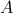has side lengths of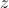. A second square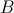has side lengths of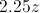. How many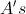can you fit in a single?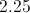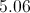Explanation:

The area ofis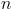, the area ofis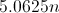. Therefore, you can fit 5.06in.

### Example Question #212 : Geometry

The perimeter of a square is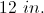If the square is enlarged by a factor of three, what is the new area?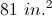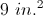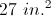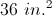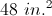Explanation:

The perimeter of a square is given by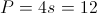so the side length of the original square is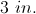The side of the new square is enlarged by a factor of 3 to give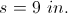So the area of the new square is given by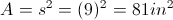.

### Example Question #36 : Squares

How much more area does a square with a side of 2r have than a circle with a radius r?  Approximate  π by using 22/7.

1/7 square units

6/7 square units

12/14 square units

4/7 square units

6/7 square units

Explanation:

The area of a circle is given by A = πr2 or 22/7r2

The area of a square is given by A = s2 or (2r)2 = 4r2

Then subtract the area of the circle from the area of the square and get 6/7 square units.

### Example Question #37 : Squares

If the perimeter of a square is 44 centimeters, what is the area of the square in square centimeters?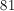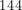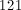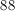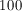Explanation:

Since the square's perimeter is 44, then each side is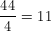.

Then in order to find the area, use the definition that the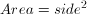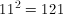### Example Question #38 : Squares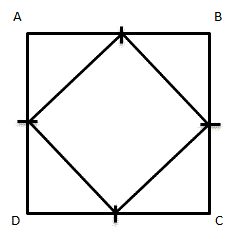Given square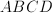, with midpoints on each side connected to form a new, smaller square.  How many times bigger is the area of the larger square than the smaller square?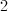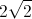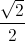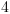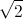Explanation:

Assume that the length of each midpoint is 1.  This means that the length of each side of the large square is 2, so the area of the larger square is 4 square units.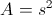To find the area of the smaller square, first find the length of each side.  Because the length of each midpoint is 1, each side of the smaller square is(use either the Pythagorean Theorem or notice that these right trianges are isoceles right trianges, so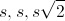can be used).

The area then of the smaller square is 2 square units.

Comparing the area of the two squares, the larger square is 2 times larger than the smaller square.

### Example Question #91 : Quadrilaterals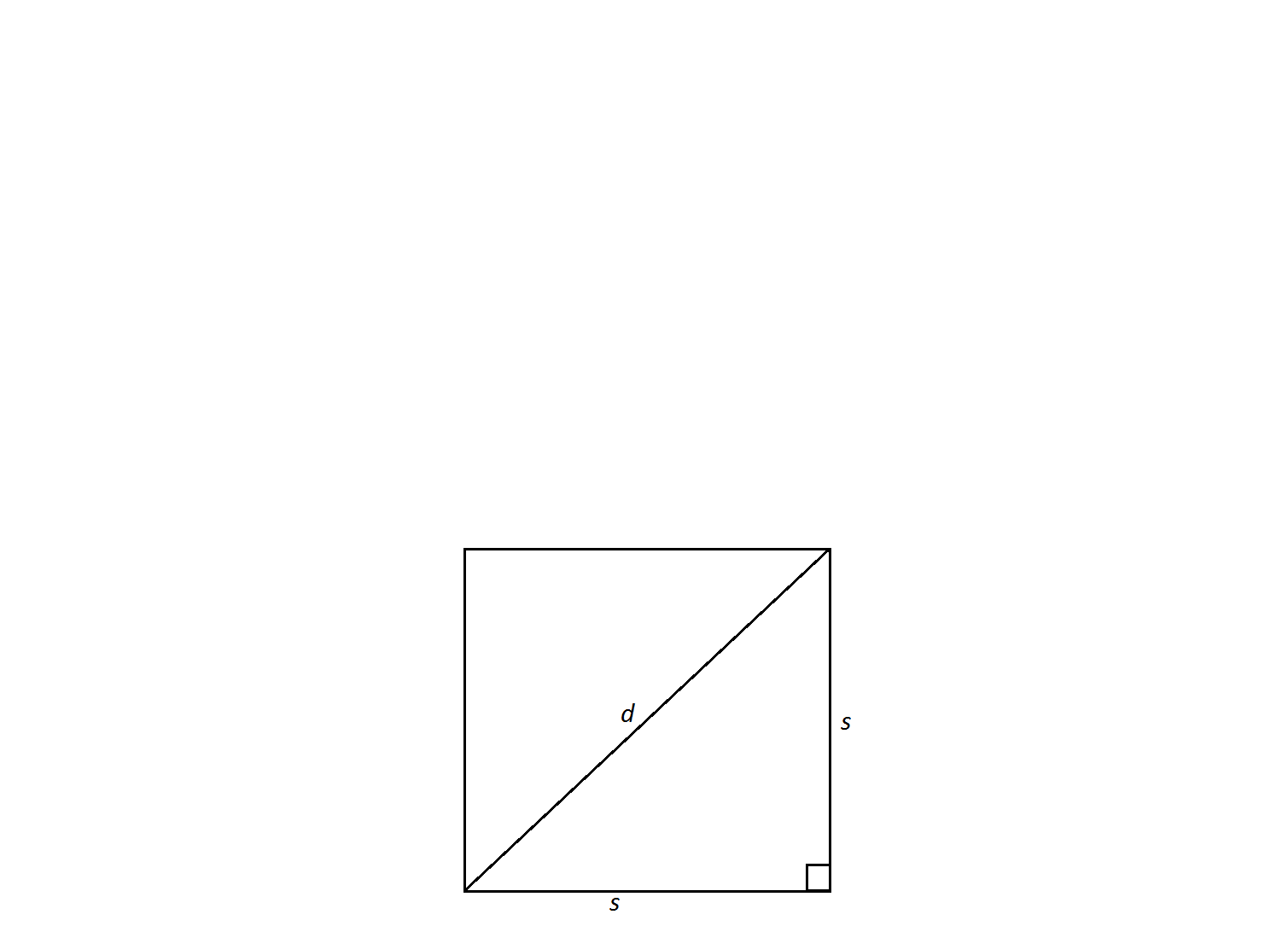Find the area of a square with a diagonal of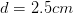.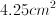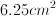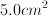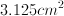Not enough information to solveExplanation:

A few facts need to be known to solve this problem. Observe that the diagonal of the square cuts it into two right isosceles triangles; therefore, the length of a side of the square to its diagonal is the same as an isosceles right triangle's leg to its hypotenuse: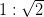.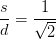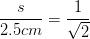Rearrange an solve for.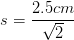Now, solve for the area using the formula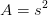.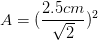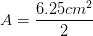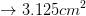### Example Question #92 : Quadrilaterals

If the ratio of the sides of two squares is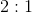, what is the ratio of the areas of those two squares?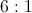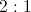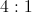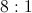Explanation:

Express the ratio of the two sides of the squares as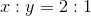. The area of each square is one side multiplied by itself, so the ratios of the areas would be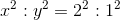. The right side of this equation simplifies to a ratio of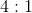.

### Example Question #39 : Squares

If a completely fenced-in square-shaped yard requires 140 feet of fence, what is the area, in square feet, of the lot?

1225

4900

140

35

70

1225

Explanation:

Since the yard is square in shape, we can divide the perimeter(140ft) by 4, giving us 35ft for each side. We then square 35 to give us the area, 1225 feet.

### Example Question #93 : Quadrilaterals

What is the area of a square with a diagonal of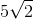?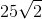Explanation:

The formula for the area of a square is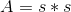. However, the problem gives us a diagonal and not a side.

Remember that all sides of a square are equal, so the diagonal cuts the square into two equal triangles, each a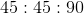right triangle.

If we use the Pythagorean Theorem, we see: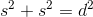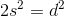Plug in our given diagonal to solve.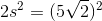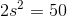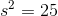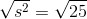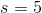From here we can plug our answer back into our original equation: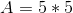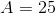### Example Question #11 : Squares

A half circle has an area of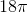. What is the area of a square with sides that measure the same length as the diameter of the half circle?

81

72

144

108

36

144

Explanation:

If the area of the half circle is, then the area of a full circle is twice that, or.

Use the formula for the area of a circle to solve for the radius:

36π = πr2

r = 6

If the radius is 6, then the diameter is 12. We know that the sides of the square are the same length as the diameter, so each side has length 12.

Therefore the area of the square is 12 x 12 = 144.